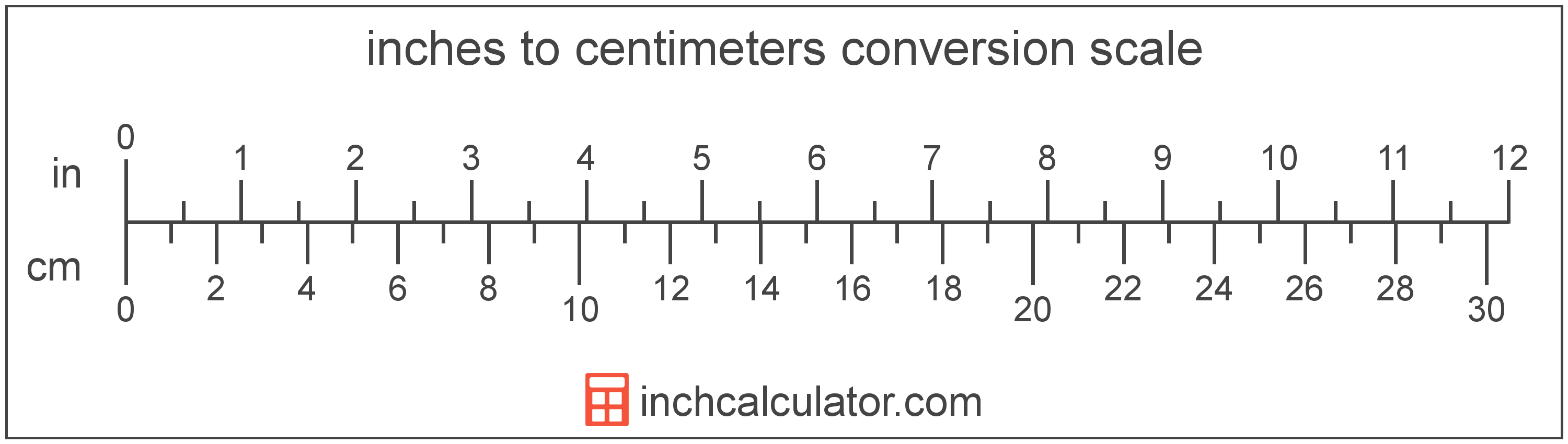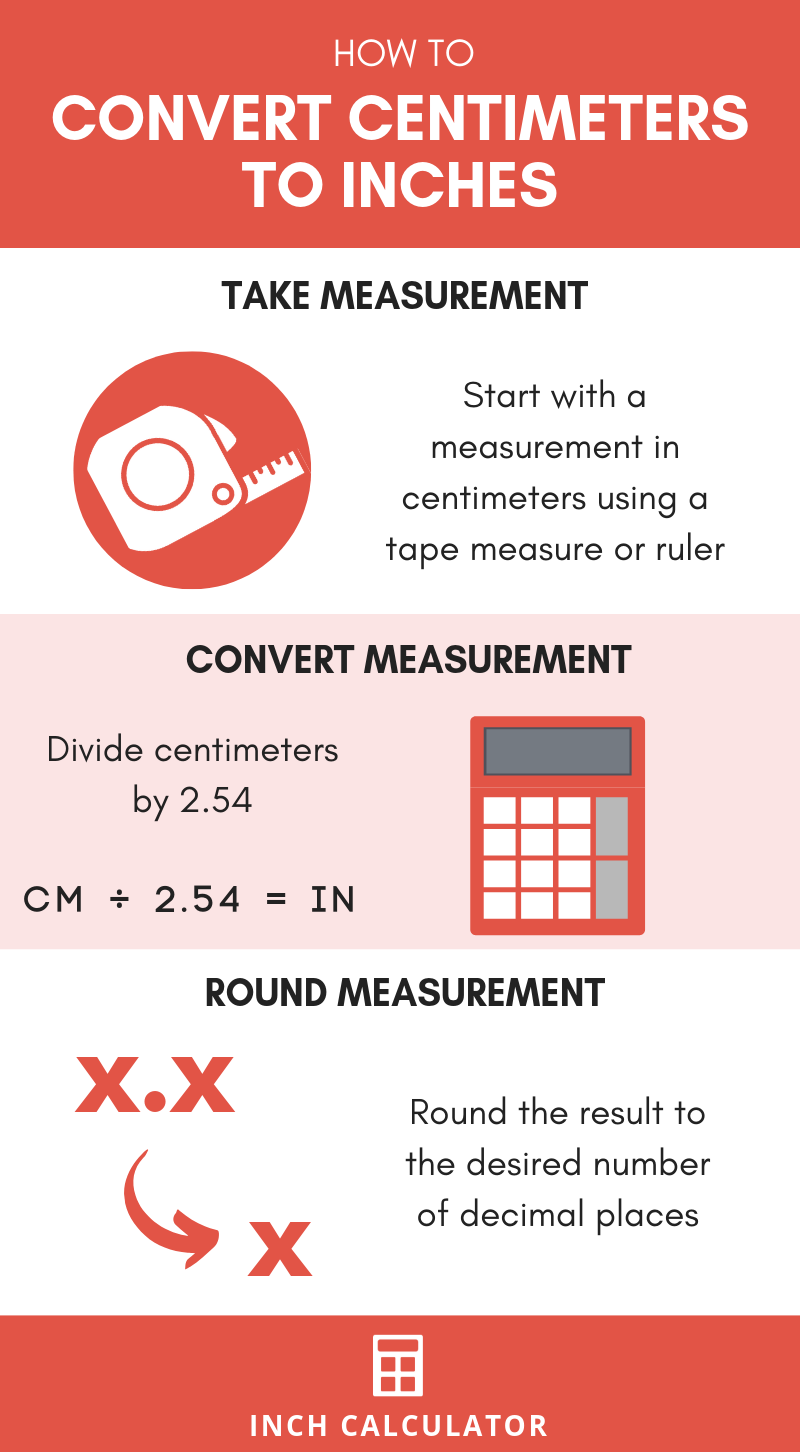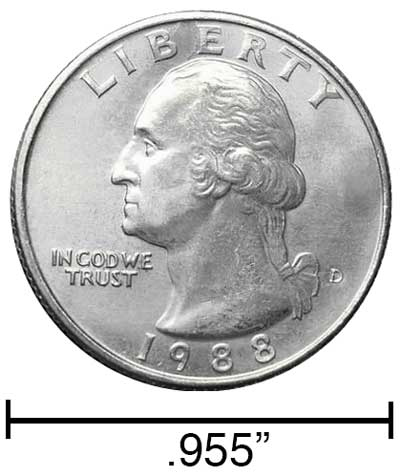# Centimeters to Inches Conversion Calculator

Enter the length in centimeters below to get the value converted to inches.

Results in Inches:1 cm = 0.393701 in
1 cm ≈ 25/64"
*inch fraction is rounded to nearest 1/64"

Do you want to convert inches to cm?

## How to Convert Centimeters to Inches

To convert a measurement in centimeters to a measurement in inches, you can use a simple formula. Since one inch is equal to 2.54 centimeters, the formula used to convert is:

inches = centimeters ÷ 2.54

The length in inches is equal to the length in centimeters divided by 2.54.

For example, here's how to convert 5 centimeters to inches using the formula above.
inches = (5 cm ÷ 2.54) = 1.968504"### How Many Inches Are in a Centimeter?

There are 0.393701 inches in a centimeter, which is why we use this value in the formula above.

1 cm = 0.393701"

### How to Convert Centimeters to Fractional Inches

We covered how to convert centimeters to inches, but you may still be wondering how to convert centimeters to fractional inches.

To do so, begin by converting cm to inches using the conversion formula above to get the resulting decimal, and then round to the nearest fractional inch. The number to the left of the decimal is the number of whole inches, and the numbers to the right of the decimal are what will be rounded to the nearest fractional inch.

To round, take the number to the right of the decimal point from the decimal result, and multiply it by the level of precision you need. You can round to the nearest 1/16, 1/32, or 1/64 of an inch.

To round to the nearest 1/16 of an inch, for instance, take the decimal portion and multiply it by 16. Then, round that result to the nearest whole number to get the numerator of the fractional inch measurement in 16ths of an inch.

For example, let's convert 3 cm to fractional inches with 1/16” precision following these steps.

Step 1: Divide 3 by 2.54 to convert cm to inches in decimal form.

3 cm ÷ 2.54 = 1.1811 in

Step 2: Multiply the decimal remainder by 16.

0.1811 × 16 = 2.8976

Step 3: Round this value to the nearest whole number; in this case, 2.8976 can be rounded to 3. Place this value in a fraction over 16, so 3/16 is the remaining fraction.

Step 4: Put it all together by placing the whole number from the decimal form in front of the fraction.

3 cm ≈ 1 3/16"

Note that we now use the "approximately equal" sign above since we rounded, and thus 3 cm is not exactly equal to 1 and 3/16 inches.

Our inch fraction calculator can add centimeters and inches together, and it also automatically converts the results to US customary, imperial, and SI metric values.## What Is a Centimeter?

One centimeter is equal to one-hundredth (1/100) of a meter, which is defined as the distance light travels in a vacuum in 1/299,792,458 of a second. One centimeter is equal to 0.393701 inches.

The centimeter, or centimetre, is a multiple of the meter, which is the SI base unit for length. In the metric system, "centi" is the prefix for hundredths, or 10-2. Centimeters can be abbreviated as cm; for example, 1 centimeter can be written as 1 cm.

Metric rulers typically have 30 cm, which are represented by 30 large tick marks. To get a rough idea of the actual length of a centimeter, a standard number 2 pencil is just about 1 cm thick.

## What Is an Inch?

An inch is a unit of length equal to 1/12 of a foot or 1/36 of a yard. Because the international yard is legally defined to be equal to exactly 0.9144 meters, one inch is equal to 2.54 centimeters.

The inch is a US customary and imperial unit of length. Inches can be abbreviated as in; for example, 1 inch can be written as 1 in.

Inches can also be denoted using the symbol, otherwise known as a double-prime. Often a double-quote (") is used instead of a double-prime for convenience. A double-prime is commonly used to express 1 in as 1″.The standard ruler is 12 inches long and is a common tool for measuring a length in inches. Another frequently used tool to perform measurements in inches is a tape measure, which commonly comes in lengths from 6' - 35'. Other types of devices used to make measurements in inches include scales, calipers, measuring wheels, micrometers, yardsticks, and even lasers.

We recommend using a ruler or tape measure for measuring length, which can be found at a local retailer or home center. Rulers are available in imperial, metric, or a combination of both values, so make sure you get the correct type for your needs.

Need a ruler? Try our free downloadable and printable rulers, which include both imperial and metric measurements.

## cm to Inches Conversion Chart

Chart of centimeter measurements converted to inches and fractional inches rounded to the nearest 1/64"
Centimeters Inches (decimal) Inches (fraction)
1 cm 0.393701" 25/64"
2 cm 0.787402" 25/32"
3 cm 1.1811" 1 3/16"
4 cm 1.5748" 1 37/64"
5 cm 1.9685" 1 31/32"
6 cm 2.3622" 2 23/64"
7 cm 2.7559" 2 3/4"
8 cm 3.1496" 3 5/32"
9 cm 3.5433" 3 35/64"
10 cm 3.937" 3 15/16"
11 cm 4.3307" 4 21/64"
12 cm 4.7244" 4 23/32"
13 cm 5.1181" 5 1/8"
14 cm 5.5118" 5 33/64"
15 cm 5.9055" 5 29/32"
16 cm 6.2992" 6 19/64"
17 cm 6.6929" 6 11/16"
18 cm 7.0866" 7 3/32"
19 cm 7.4803" 7 31/64"
20 cm 7.874" 7 7/8"
21 cm 8.2677" 8 17/64"
22 cm 8.6614" 8 21/32"
23 cm 9.0551" 9 1/16"
24 cm 9.4488" 9 29/64"
25 cm 9.8425" 9 27/32"
26 cm 10.24" 10 15/64"
27 cm 10.63" 10 5/8"
28 cm 11.02" 11 1/32"
29 cm 11.42" 11 27/64"
30 cm 11.81" 11 13/16"
31 cm 12.2" 12 13/64"
32 cm 12.6" 12 19/32"
33 cm 12.99" 12 63/64"
34 cm 13.39" 13 25/64"
35 cm 13.78" 13 25/32"
36 cm 14.17" 14 11/64"
37 cm 14.57" 14 9/16"
38 cm 14.96" 14 61/64"
39 cm 15.35" 15 23/64"
40 cm 15.75" 15 3/4"

## References

1. National Institute of Standards and Technology, Checking the Net Contents of Packaged Goods, Handbook 133 - 2019 Edition, https://nvlpubs.nist.gov/nistpubs/hb/2019/NIST.HB.133-2019.pdf